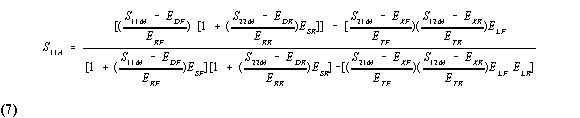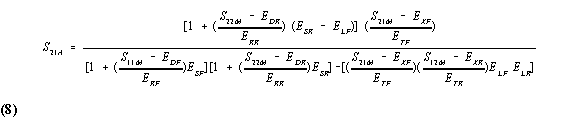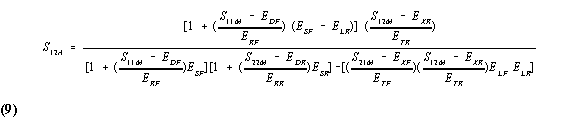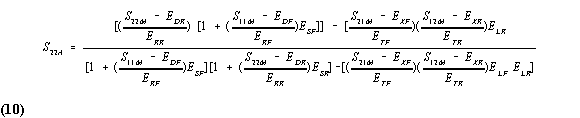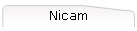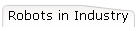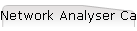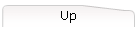# Network Analyser Calibration

Network analysers effectively compare the power of signals transmitted through, or reflected back from a network port to the power of the incident signal present at the input of that port. A combination of directional couplers, isolators, transmission lines and computer calculation is used to achieve this.

A perfect measurement system would have infinite dynamic range, isolation, and directivity characteristics, no impedance mismatches in any part of the test setup, and a flat frequency response. Although modern microwave devices for inclusion in an analyser system can be made to posses excellent response characteristics, there will inevitably be mismatches and errors in the system. We model the network analyser system response to a 12 term error model in which all possible error coefficients, their direction and position in the system are allocated.

By employing a standard calibration procedure, the individual error terms can be calculated, so reducing the uncertainty in a given measurement system. In practice, this 'perfect' network analyser is achieved by measuring the magnitude and phase response of known standard devices, using this data in conjunction with the model of the measurement system to determine error contributions, then measuring a test device and using vector mathematics to compute the actual test device response by removing the error terms.

Such a calibration routine must be repeated each and every time a part of the test setup is altered. Changing connector types, frequency ranges, system impedances, length of transmission line all contribute some alteration in impedance or phase response of the system, and so must be characterised out to remove uncertainty due to these factors.

The dynamic range and accuracy of the measurement is the limited by system noise and accuracy to which the characteristics of the calibration standards are known. This is the basic concept of vector accuracy enhancement.

10.2.1 SOURCES OF MEASUREMENT ERRORS

Network analysis measurement errors can be separated into two categories:

Random Errors. These are non-repeatable measurement variations that occur due to noise, environmental changes and other physical changes in the test setup between calibration and measurement. These are any errors that the system itself cannot measure or cannot model with an acceptable degree of certainty.

Systematic Errors. These are repeatable errors. They include mismatch and leakage terms in the test setup, isolation characteristics between the reference and test signal paths, and system frequency response.

Thus, any measurement result is the vector sum of the actual test device response plus all error terms. The precise effect of each error term depends upon its magnitude and phase relationship tot he actual test device response. Random errors cannot be precisely quantified, so they must be treated as producing a cumulative ambiguity in the measured data.

It is fortunate that in the case of most microwave measurements, systematic errors are the ones which produce the most significant measurement uncertainty. Since each of these errors produces a predictable effect upon the measured data, their effects can be removed to obtain a corrected value for test device response. For the purpose of vector accuracy enhancement, these uncertainties are quantified as directivity, source match, load match, isolation and tracking (frequency response). When accuracy enhancement techniques are used, the resultant values after correction are termed Effective Directivity, Effective Source and Load Match, Effective Isolation, and Effective Tracking.

10.2.2 DIRECTIVITY

This is defined as the vector sum of all leakage signals appearing at the network analyser input due to the inability of the signal separation device to absolutely separate incident and reflected waves, as well as residual reflection effects of test cables and adapters between the signal separation device and the measurement plane. The uncertainty contributed by directivity is independent of the characteristics of the test device and it usually produces the major ambiguity in reflection measurements.

The standard test sets typically provide uncorrected directivity of greater than 26dB. However, the vector accuracy enhancement technique described here will typically produce much greater Effective Directivity.

10.2.3 SOURCE MATCH

This is defined as the vector sum of signals appearing at the network analyser test input due to the inability of the source to maintain absolute constant power at the test device input as well as cable and adapter mismatches and losses outside the source levelling loop. The uncertainty contributed by source match is dependent upon the relationship between the actual input impedance of the test device and the equivalent match of the source, and it is a factor in both transmission and reflection measurements.

Source match error is particularly a problem when measuring very high or very low impedances (large mismatch at the measurement plane). The Effective Source Match can be improved considerably by using vector error correction techniques.

Load match is defined as the vector sum of signals appearing at the network analyser test input due to effects of impedance mismatches between the test device output port and the network analyser test input. The uncertainty contributed by Load Match is dependent upon the relationship between the actual output impedance of the test device and the effective match of the return port, and is a factor in all transmission measurements and in reflection measurements of two-port devices.

Load match effects are analyzed similarly to source match effects and will produce major transmission measurement errors for a test device whose output port is highly reflective. Effective Load Match can typically by improved by a factor of 15 to 20dB using vector accuracy enhancement techniques.

10.2.5 ISOLATION

This is the vector sum of signals appearing at the network analyser detectors due to crosstalk between the reference and test signal paths, including signal leakage within both the RF and IF sections of the receiver. The uncertainty contributed by isolation is a factor for concern in high loss transmission measurements.

The system typically maintains greater than 80dB of isolation between the reference and test signal paths. Characterisation and removal of repeatable crosstalk and leakage, along with extensive averaging, improves the Effective Isolation to extend the measurement system dynamic range by up to 20dB.

10.2.6 TRACKING

This is the vector sum of all test setup variations in magnitude and phase frequency response, including signal separation device, test cables and adapters, and variations in frequency response between the reference and test signal paths. This error is a factor in both transmission and reflection measurements.

The magnitude and phase/frequency response variations and the resultant measurement errors are reduced using vector accuracy enhancement, making the Effective Tracking typically under 0.02dB and 0.1 degree.

10.3 CORRECTING MEASUREMENT ERRORS

The HP8510B network analyser system offers a choice between Frequency-Response-Only, 1-Port, and 2-Port error models. The frequency-response-only error model provides signal path frequency response error correction for the selected parameter. This model may be adequate for measurement of well matched low loss devices where vector normalisation of magnitude and phase frequency response errors provides sufficient measurement accuracy.

The 1-Port error model provides directivity, source match, and reflection signal path frequency response vector error correction for reflection measurements. This model is best applied to high accuracy reflection measurements of one-port devices.

The 2-Port error model provides full directivity, isolation, source match, load match and frequency response vector error correction for transmission and reflection measurements of two-port devices. This model provides best magnitude and phase measurement accuracy for two-port devices but requires measurement of all four S-parameters of the two-port device.

The following text describes these error models in greater detail, and, more importantly, explains how they can be characterised and used to reduce measurement uncertainty. In actual system operation, error correction is not always done exactly as suggested, but the results are mathematically equivalent.

Let us consider measurement of reflection coefficients (magnitude and phase) of some unknown one-port device. No matter how careful we are, the measured data will differ from the actual. Directivity, Source Match, and Tracking are the main sources of error.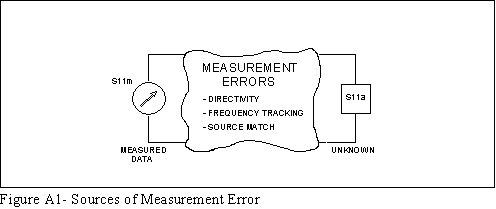Reflection coefficient is measured by first separating the incident voltage wave (I) from the reflected voltage wave (R), then taking the ratio of the two values. Ideally, (R) consists only of the wave actually reflected by the test device (S11a).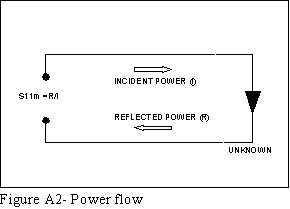Unfortunately, not all of the incident wave reaches the unknown. Some of (I) may appear at the measurement system input due to leakage through the signal separation device (coupler/bridge). Also, some of (I) may be reflected by imperfect adapters between signal separation device and the measurement plane. The vector sum of the leakage and miscellaneous reflections is directivity, EDF. Understandably, out measurement is distorted when the directivity signal combines vectorially with the actual reflected signal from the unknown S11a.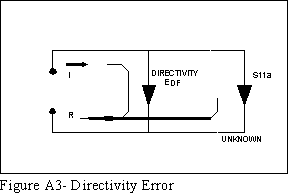Since the measurement system test port is never exactly the characteristic impedance (normally 50 ohms), some of the reflected signal bounces off the test port (or other impedance changes further down the line) and back to the unknown, adding to the original incident signal (I). This effect causes the magnitude and phase of the incident signal to vary as a function of S11a. Levelling the source to produce constant (I) reduces this error, but since the source cannot be levelled exactly at the test device input, levelling cannot eliminate all power variations. This re-reflection effect and the resultant incident power variation is caused by the source match error, ESF.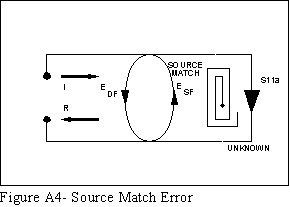Tracking (frequency response) error is caused by variations in magnitude and phase flatness versus frequency between the test and reference signal paths. These are due mainly to imperfectly matched samplers and difference between reference and test signal paths. The vector sum of these variations is the reflection signal path tracking error, ERF.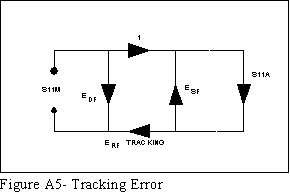It can be shown that these three errors are mathematically related to the actual, S11a, and measured S11m, data by the following equation.If we knew the value of these three 'E' errors and the measured test device response at each frequency step, we could simply solve the above equation for S11a to obtain the actual frequency response. Because each of these errors changes with frequency, it is necessary that their values by known at each test frequency. They are found by measuring (calibrating) the system at the measurement plane using three independent standards whose S11a is known at all frequencies.

One of the standards we apply is a 'perfect' load which makes S11a=0 and essentially measures directivity. By 'perfect' load we mean a reflectionless termination at the measurement plane. All incident energy is absorbed. With S11a = 0 the equation can be solved for EDF, the directivity error term. Of course, in practice, the perfect load can never be achieved.Since the measured value for directivity is the vector sum of the actual directivity plus the actual reflection coefficient of the 'perfect' load, any reflection from the termination represents an error. System Effective Directivity becomes the actual reflection coefficient of the 'perfect' load. In general, any termination having a return loss greater than the uncorrected system directivity reduces reflection measurement uncertainty.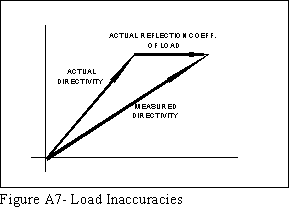Due to the difficulty of producing a high quality fixed coaxial termination at microwave frequencies, a sliding load can be used at each test frequency to separate the reflection of a somewhat imperfect termination from the actual directivity.

At any single frequency, moving the sliding termination with respect to the measurement plane produces a complete circle when the sliding element is displaced on-half wavelength of the test frequency. Its reflection coefficient magnitude remains constant but the phase of the coefficient changes. The radius of that circle is the actual reflection coefficient of the sliding termination, and the centre of the circle is terminated by the actual directivity of the test setup and the geometry of the air line within the sliding load.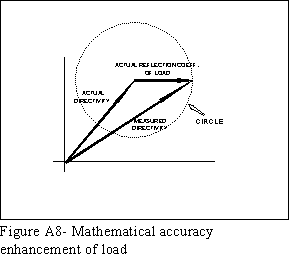Thus the critical specifications for the sliding load assembly are the mechanical dimensions (impedance) of the connector, of the transmission line between the measurement plane and the termination, and that the termination maintains a constant reflection coefficient magnitude at all positions.

The sliding load calibration sequence used here measures the sliding load at 6 or more positions. The centre of the circle is calculated by the HP8510B firmware, which may be a 20dB improvement over a fixed load. A problem arises in the use of the sliding load at low microwave frequencies. The line has to be very long, otherwise the points are bunched together and it is very difficult to determine the centre accurately. In these cases it is often better to use a fixed load. The convention is to use a fixed load below 2GHz and a sliding load above 2GHz.

Next a short circuit termination is used to establish another condition.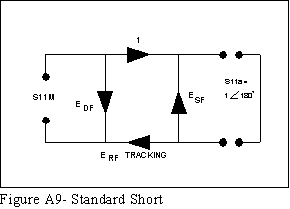The open circuit gives us the third independent condition. Now values for EDF, directivity, ESF, source match, and ERF, reflection tracking, are computed and stored.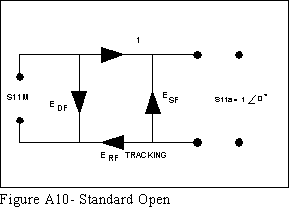Now we measure the unknown to obtain a value for the measured response, S11m, at each frequency.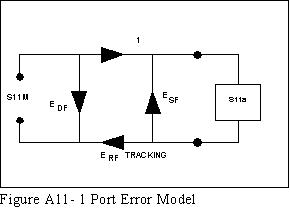This is the 1-port error model equation solved for S11a. Since we have the three errors and S11m for each test frequency, we can now compute S11a.For reflection measurements on two-port devices, the same technique can be applied, but the test device output port must be terminated in the system characteristic impedance. This termination should be at least as good (low reflection coefficient) as the load used to determine directivity. The additional reflection error caused by an improper termination at the test device output port is not incorporated into the 1-port error model.

10.4 2-PORT MODEL

Now consider measurement of transmission coefficients (magnitude and phase) of an unknown two-port device. The major sources of error are Tracking, Source Match, Load Match, and Isolation. These errors are reduced or eliminated using the 2-port error model.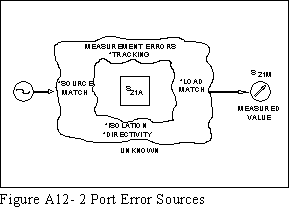Transmission coefficient is measured by taking the ratio of the incident voltage wave (I) and the transmitted wave (T). Ideally, (I) consists only of power delivered by the source and (T) consists only of power emerging at the test device output.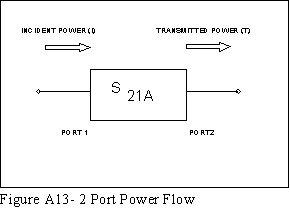As for the reflection model, source match can cause the incident signal to vary as a function of test device S11A. Also, since the test setup transmission return port is never exactly the characteristic impedance, some of the transmitted signal is reflected from the test set port 2, and other mismatches between the test device output and the detector, to return to the test device. A portion of this wave may be re-reflected at port 2, thus affecting S21M, or part may be transmitted through the device in the reverse direction to appear at port 1, thus affecting S11M. This error term, which causes the magnitude and phase of the transmitted signal to vary as a function of S22A, is called load match, ELF.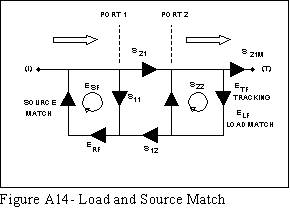The measured value S21M, consists of wave components which vary as a function of the relationship between ESF and S11A as well as ELF and S22A, so the input and output reflection coefficients of the test device must be measured and stored for use in the S21A error correction calculation. Thus, the test setup is calibrated as described above for reflection to establish directivity, EDF, source match, ESF, and reflection tracking, ERF, terms for the reflection measurements.

Since we now have a calibrated port for reflection measurements, we connect the thru' and determine load match, ELF by measuring the reflection coefficient of the thru connection.

Transmission tracking is the measured with the thru connection. The data is corrected for source and load match effects, and stored as transmission tracking, ETF.

Isolation, EXF, represents the part of the incident wave that appears at the receiver detectors without actually passing through the test device. Isolation is measured with the test set in the transmission configuration and terminations installed at the points at which the test device will be connected.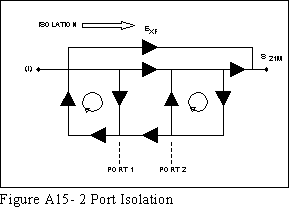The microwave test set as installed in the Bath University Microwave research laboratory (HP8515A) can measure both the forward and reverse characteristics of the test device without the need to manually remove and physically reverse it. For these test sets, the Full 2-port transmission and reflection error model shown above includes terms for:

Directivity, EDF (forward) and EDR (reverse)

Isolation, EXF and EXR

Source Match, ESF and ESR

Load Match, ELF and ELR

Transmission Tracking, ETF and ETR

Reflection Tracking, ERF and ERR

Thus there are two sets of error terms, one for each of forward and reverse, with each set consisting of six error terms.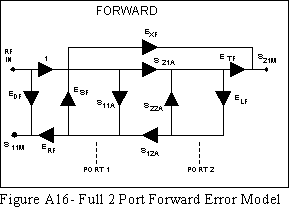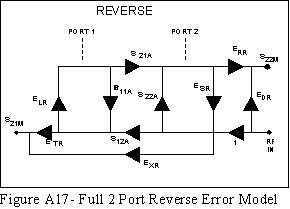If the test set cannot switch between forward and reverse directions, then the reverse terms can not be measured directly and the forward error terms are used in their place when the test device is manually reversed. The One-path 2-port error model makes this assumption.

Below are shown the 2-port error model equations for all S-parameters of a two port device. Note that the mathematics for this comprehensive model uses all forward and reverse error terms and measured values. Thus to perform full error correction, for any one parameter of a two-port device, all four S-parameters must be measured.